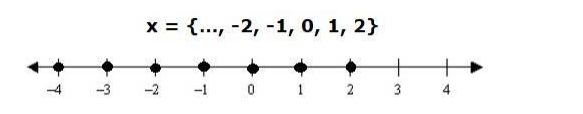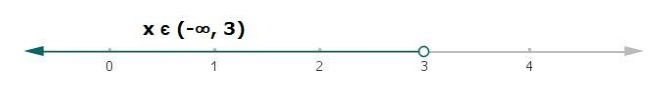# Solve each of the following in equations and represent the solution set on

Question:

Solve each of the following in equations and represent the solution set on the number line.

$5 x+2<17$, where (i) $x \in Z$, (ii) $x \in R$.

Solution:

(i) $5 x+2<17, x \in Z$

Subtracting 2 from both the sides in the above equation,

$5 x+2-2<17-2$

$5 x<15$

Dividing both the sides by 5 in the above equation,

$\frac{5 x}{5}<\frac{15}{5}$

$x<3$

Since x is an integer

Therefore, possible values of x can be

$x=\{\ldots,-2,-1,0,1,2\}$(ii) $5 x+2<17, x \in R$

Subtracting 2 from both the sides in above equation

$5 x+2-2<17-2$

$5 x<15$

Dividing both the sides by 5 in above equation

$\frac{5 x}{5}<\frac{15}{5}$

$x<3$

Therefore, $x \in(-\infty, 3)$